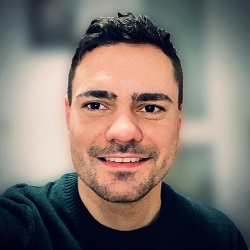Homepage of Patrik KnopfDr. Patrik Knopf   Research Assistant (Akademischer Rat a.Z.) Faculty for Mathematics University of Regensburg Universitätsstraße 31 93040 Regensburg Germany Email: patrik.knopf∂ur.de Office: M114 Phone: +49 (0)941 943 2953

This is the homepage of Patrik Knopf.

You can browse through this site here:

# Research

## Research interests:

• Partial differential equations, especially
• Phase field models (especially the Cahn-Hilliard equation) and their applications (e.g., tumor growth models)
• Kinetic equations from plasma physics (e.g., Vlasov-Poisson and Vlasov-Maxwell equations).

• Calculus of variations

• Optimal control
• Optimal control theory for problems with PDE constraints
• Shape and topology optimization via phase-field approach

• Numerical methods and simulations for PDEs
• Finite element methods
• Particle-in-cell methods for kinetic equations

## Research projects and collaborators:

• Analysis and numerical methods for the Cahn-Hilliard equation with dynamic boundary conditions with

• Analysis and numerical methods for anisotropic diffuse interface models with

• Julia Wittmann (University of Regensburg)
• Analysis and optimal control of magneto-viscoelastic fluids with

• Analysis and optimal control of phase-field models for tumor growth with

• Analysis of stratified water waves with

• Elliptic problems with bulk-surface coupling: Well-posedness, regularity theory, eigenvalue problems with

• Optimal control and confinement of plasmas modeled by Vlasov equations with

• Shape and topology optimization via multi-phase-field approach with

• Two-phase flows with bulk-surface interaction described by dynamic boundary conditions with